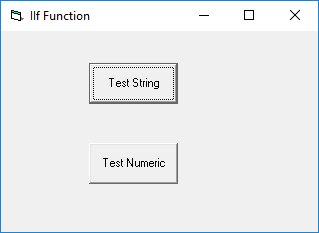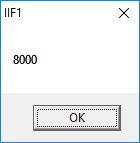# Lesson 7 If statement and IIf() Function

In previous lessons, we have learned how to create Visual Basic code that can accept input from the user and display the output without controlling the program flow. In this chapter, you will learn how to create VB code that can make a decision when it process input from the user, and control the program flow in the process. Decision making process is an important part of programming because it can help to solve practical problems intelligently so that it can provide useful output or feedback to the user. For example, we can write a  program that can ask the computer to perform certain task until a certain condition is met.

## 7.1 Conditional Operators

To control the VB program flow, we can use various conditional operators. Basically, they resemble mathematical  operators. Conditional operators are very powerful tools, they let the VB program compare data values and then decide what action to take, whether to execute a program or terminate the program and more. These operators are shown in Table 7.1.

#### Table 7.1: Conditional Operators

Operator
Meaning
= Equal to
> More than
< Less Than
> More than or equal
<= Less than or equal
<> Not Equal to

## 7.2 Logical Operators

In addition to conditional operators, there are a few logical operators that offer added power to the VB programs. They are shown in Table 7.2.

#### Table 7.2:Logical Operators

Operator Description
And Both sides must be true
Or One side or other must be true
Xor One side or other must be true but not both
Not Negates true

* You can also compare strings with the operators. However, there are certain rules to follow where upper case letters are less than lowercase letters, and number are less than letters.

## 7.3  Using  If.....Then.....Else  Statements  with Operators

To effectively control the VB program flow, we shall use If...Then...Else statement together with the conditional operators and logical operators.

```If conditions Then

VB expressions

Else

VB expressions

End If
```

### Example 7.1:

#### The Code

```Private Sub OK_Click()

End If
End Sub
```

#### The Output#### Figure 7.1#### Figure 7.2

You can check out our animated passwords cracker program

### Example 7.2

This example calculate the commission based on sales volume attained. Let's say the commission structure is laid out as in the table below:

Sale Volume(\$)Commission(%)
<5000 0
5000-99995
1000-1499910
15000-1999915
20000 and above20

In this example, we insert a textbox to accept sale volume input and a label to display commission. Insert a command button to trigger the calculation

#### The Code

```
Private Sub cmdCalComm_Click()
Dim salevol, comm As Currency
salevol = Val(TxtSaleVol.Text)
If salevol >= 5000 And salevol < 10000 Then
comm = salevol * 0.05
ElseIf salevol >= 10000 And salevol < 15000 Then
comm = salevol * 0.1
ElseIf salevol >= 15000 And salevol < 20000 Then
comm = salevol * 0.15
ElseIf salevol >= 20000 Then
comm = salevol * 0.2
Else
comm = 0
End If
LblComm.Caption = Format(comm, "\$#,##0.00")
End Sub
```

### Example 7.3: Guess a Number Game

This is a guess a number game where the user key in a number and see check whether the answer is correct. Thr program will provide a hint whether the number is too small or too big. After a number of trial, the user should get the right answer. The program employ the If..Then..Else technique to check whether the entry is correct.

##### The Code
```'Guess a Number
Const realNumber = 99
Dim userNumber As Integer

Private Sub EXit_Click()
End
End Sub

Private Sub OK_Click()

userNumber = entry.Text
If userNumber > realNumber Then

hint.Caption = "Your number is too big"
ElseIf userNumber < realNumber Then

hint.Caption = "Your number is too small"

entry.Text = ""
entry.SetFocus
Else

hint.Caption = "Congratulation, your number is correct"

End If

End Sub
```

## 7.4 The IIf() Function

The IIf function denotes immediate decision function. It provides a simple decision making process based on three arguments, as follows:

```
IIf(x, y, z)
```

x represents a logical expression while y and z represent a numeric or a string expression.

For example, the IIF(x>y, expression 1, expression 2) function evaluates the values of x and y, if x>y. then expression 1 is true, otherwise the expression 2 is true.

### Example 7.3

```Private Sub CmdNumeric_Click()
Dim x, y, a, b, ans As Double
x = InputBox("Enter a number")
y = InputBox("Enter another number")
a = Val(x)
b = Val(y)

ans = IIf(a < b, b - a, a * b)
MsgBox ans
End Sub

Private Sub CmdString_Click()
Dim A, B, C As String
A = InputBox("Enter a word")
B = InputBox("Enter another word")
C = IIf(A < B, A, B)
MsgBox C
End Sub
```#### Figure 7.4: The Interface

If you click test string and enter the first word long and the second word short, the logical condition is true, hence the word long will be displayed, as shown in Figure 7.5.#### Figure 7.5

If you click test numeric and enter the first number 200 and the second number 40, the logical condition is false, hence the second expression will be executed, which is 20x40=800, as shown in Figure 7.6.